# COS1521: Second Semester: ASSIGNMENT 01 – 2016

By | January 6, 2017
 Due date: 24-Aug-16 Study material: (1) F&M: chapters 1–4; Appendices A & E (prescribed book) (2) Tutorial Letter 102: Part I: units 1–4 Part II: ALL (pages 24–74) Part III: solution to self-assessment assignment

Do the relevant exercises in F&M, Tutorial Letter 102 and the self-assessment assignment before attempting this assignment. See more information about Tutorial Letter 102 in section 2.3 of this tutorial letter.

Submission procedure: Via myUnisa (see section 8.4)

Contribution to semester mark: 40%

Unique assignment number: 703201

This assignment is compulsory. To be admitted to the examination for this module, you must submit this assignment by 24 August 2016.

•  Each multiple-choice question has four possible answers. Select the alternative you consider to be the most appropriate.
• Submit your assignment via myUnisa by the due date. Do not be concerned if myUnisa is down on the due date. If this happens, we are notified about the situation and will take this type of delay into consideration. Try to resubmit the assignment as soon as myUnisa is up again.
• The mark that you achieve out of a possible 40 marks will be converted to a percentage and will contribute 40% towards your semester mark.

Second semester: Assignment 01

QUESTION 1

According to the von Neumann model, which subsystem of a computer serves as a manager of other subsystems?

1. ALU
2. Input/output
3. Control unit
4. Memory

ANS: 3

Page reference in F&M: 5

QUESTION 2

We divide computer software and hardware into generations. In which generation are we currently?

1. Sixth
2. Third
3. Fifth
4. Fourth

ANS: 3

Page reference in F&M: 11

QUESTION 3

Which of the given options is not true? Since the 1950s, computers have generally become …

1. faster.
2. smaller.
3. cheaper.
4. heavier.

ANS: 4

Page reference in F&M: 10

QUESTION 4

Before cell phones (mobile phones) emerged, some people used to communicate using e-mail and others sent mail through the post office (snail mail). However, this communication gap has narrowed with the use of SMSs sent via cell phones. What name is given to the situation described here?

1. Dependency
2. Social justice
3. Digital divide
4. Privacy

ANS: 3

Page reference in F&M: 11

QUESTION 5

What name is given to a set of instructions that tells the computer what to do with data?

1. A program
2. An algorithm
3. A data processor
4. An operating system

ANS: 1

Page reference in F&M: 2

QUESTION 6

The first electronic special-purpose computer was called …

1. ABC
2. Pascaline
3. ENIAC
4. Pascal

ANS: 1

Page reference in F&M: 9

QUESTION 7

The area involving the design and writing of structured programs that follow strict rules and principles is known as …

1. software engineering.
2. application development.
3. algorithm design.
4. program development.

ANS: 1

Page reference in F&M: 8

QUESTION 8

Convert (10101)2 to a decimal number.

1. (5)10
2. (21)10
3. (17)10
4. (33)10

ANS: 2

Page reference in F&M: 26

QUESTION 9

Convert (B3.2)16 to an octal number.

1. (83.2)8
2. (263.2)8
3. (563.2)8
4. (263.1)8

ANS: 4

Page reference in F&M: 33

QUESTION 10

Which one of the following number representations is not correct?

1. (111.1)10
2. (12.01)2
4. (417)8

ANS: 2

Page reference in F&M: 25

QUESTION 11

Convert (34)10 to a hexadecimal number.

1. (34)16
2. (22)16
3. (24)16
4. (42)16

ANS: 2

Page reference in F&M: 30

QUESTION 12

Convert (22)16 to a binary number.

1. (1111)2
2. (1010)2
3. (100010)2
4. (111100)2

ANS: 3

Page reference in F&M: 32

QUESTION 13

Which of the following is false?

1. (0)10 = (0)2
2. (10)8 is less than (10)10
3. (10)16 is an even number
4. (F)16 is equal to (14)10

ANS: 4

Page reference in F&M: 23-25

QUESTION 14

What is the 2’s complement representation of −14 using 5 bits?

1. (11110)2
2. (10100)2
3. (11001)2
4. (10010)2

ANS: 4

Page reference in F&M: 50-51

QUESTION 15

Which one of the following statements regarding sign-and-magnitude representation is not true?

1. There are two 0s in sign-and-magnitude representation: positive zero and negative zero.
2. Sign-and-magnitude representation is often used to quantise an analog signal.
3. Sign-and-magnitude numbers are subject to positive and negative overflow.
4. Sign-and-magnitude representation is commonly used to store integers.

ANS: 4

Page reference in F&M: 64

QUESTION 16

Samples are taken if all the values of an audio signal cannot be recorded. The number of samples needed to retrieve a replica of the original image depends on …

1. quantization.
2. encoding.
3. the bit depth.
4. the maximum number of changes in the analog signal.

ANS: 4

Page reference in F&M: 65

QUESTION 17

Which one of the following statements regarding the storing of audio or images is not true?

1. Vector graphics are suitable for storing the fine details of photographic images.
2. Audio storing can involve sampling, quantization and encoding.
3. In 2014, the dominant standard for storing audio was MP3.
4. Raster graphics are used when an analog image such as a photograph must be stored.

ANS: 1

Page reference in F&M: 67, 68

QUESTION 18

How many bytes should be used for a 32-bit pattern?

1. 4
2. 5
3. 16
4. 32

ANS: 1

Page reference in F&M: 43

QUESTION 19

If the input is 1010011, and the mask 0011111 is used to unset the input, what is the resulting output?

1. 0000000
2. 1111111
3. 0010011
4. 0000011

ANS: 3

Page reference in F&M: 81, 82

QUESTION 20

Calculate: (11011)2 + (1001)2.

1. (100100)2
2. (100010)2
3. (111000)2
4. (101100)2

ANS: 1

Page reference in F&M: 87

QUESTION 21

Calculate: (101011.1)2 + (11001.01)2.

1. (1110010.10)2
2. (1000010.01)2
3. (10010011.11)2
4. (1000100.11)2

ANS: 4

Page reference in F&M: 87

QUESTION 22

Calculate: (1010)2 XOR (1001)2.

1. (0011)2
2. (0110)2
3. (0111)2
4. (1111)2

ANS: 1

Page reference in F&M: 81

QUESTION 23

Use the arithmetic left shift operation on the bit pattern 11001101. The pattern is an integer in two’s complement format.

1. (10011010)2
2. (11001101)2
3. (11000110)2
4. (11100101)2

ANS: 1

Page reference in F&M: 85

QUESTION 24

Which logical operation on two input patterns has the following property?

“If a bit in one input is 0, there is no need to check the corresponding bit in the other input. The result is always 0.”

1. AND
2. OR
3. XOR
4. NOT

ANS: 1

Page reference in F&M: 79

Apply Boolean algebra rules to determine the simplest forms of the given Boolean functions in the following THREE questions.

QUESTION 25

What is the simplest form of the Boolean function (xx’ + y)’?

1. y’
2. 0
3. (x + x’)y’
4. 1

ANS: 1

QUESTION 26

What is the simplest form of the Boolean function x + xy’z’ + xy’z’?

1. y’z’
2. xy’z’
3. x
4. x + xy’z’

ANS: 3

QUESTION 27

What is the simplest form of the Boolean function (x’y)’ + y?

1. x + y
2. xy’ + y
3. 1
4. Y

ANS: 3

QUESTION 28

Consider the following Boolean function:
F(x,y,z) = m2 + m4 + m5 + m6

Which one of the following four Karnaugh diagrams represents the given function?

1.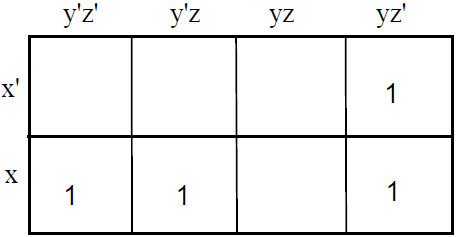2.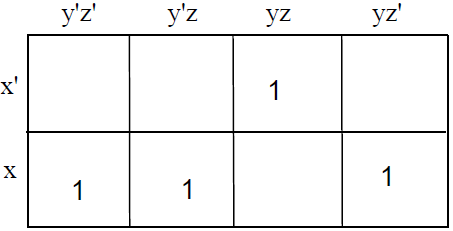3.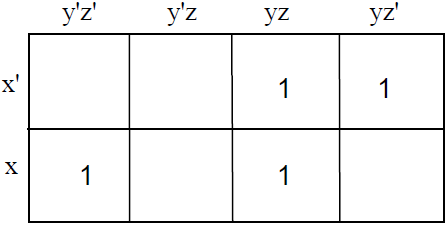4.ANS: 1

QUESTION 29

Consider the following Karnaugh map:Which one of the following four Karnaugh maps reflects the correct forming of groups?

1.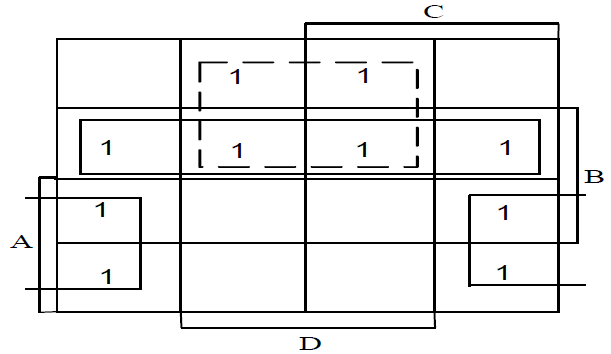2.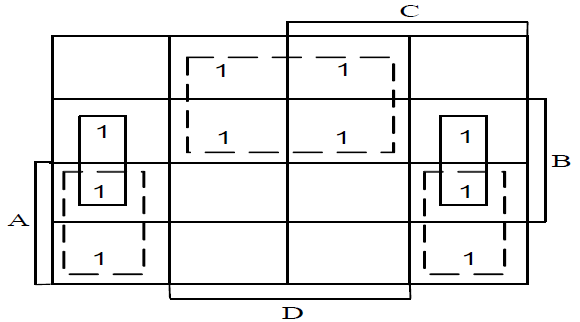3.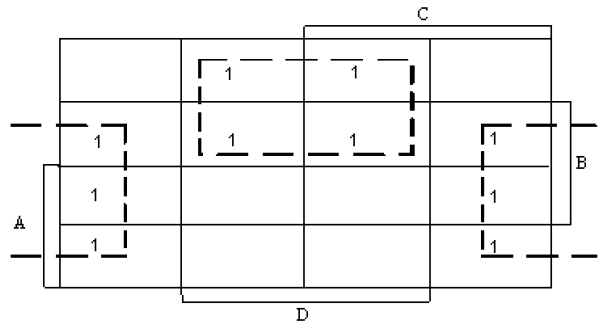4.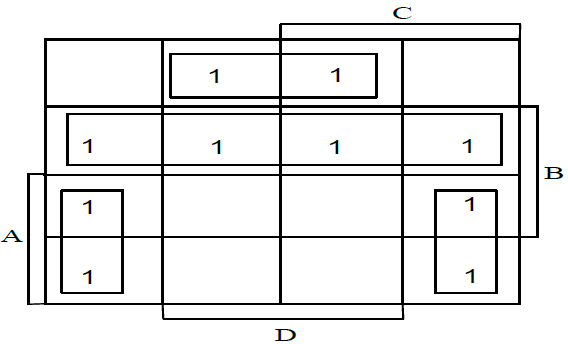ANS: 1

The next THREE questions refer to the Karnaugh map below.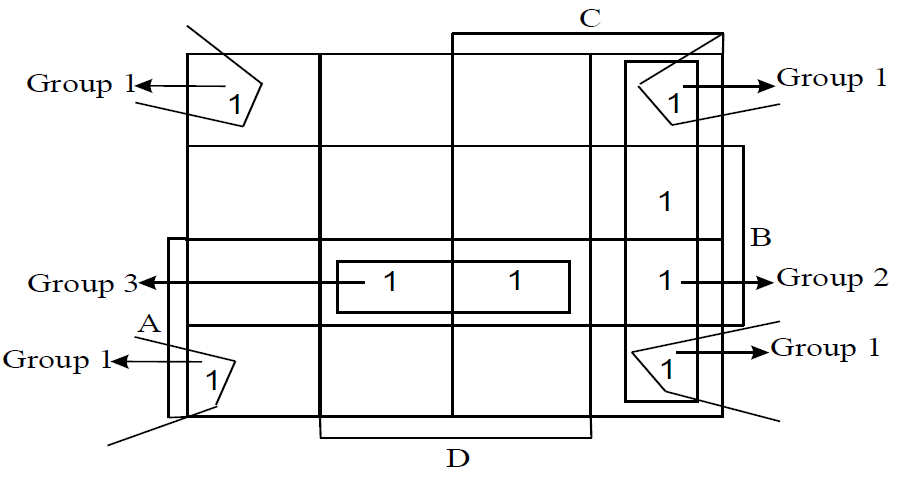QUESTION 30

Which term represents Group 1?

1. A’B’CD’
2. D’
3. A’
4. B’D’

ANS: 4

QUESTION 31

Which term represents Group 2?

1. DC’
2. C’
3. D’
4. CD’

ANS: 4

QUESTION 32

Which term represents Group 3?

1. ABD
2. AB
3. D
4. ACD

ANS: 1

The next FOUR questions refer to the following combinational logic circuit: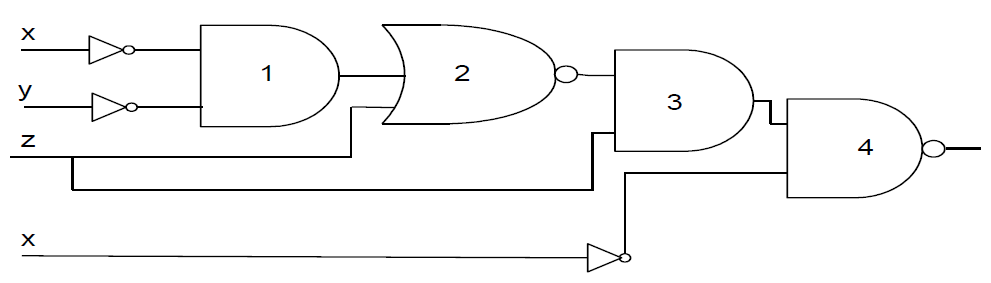QUESTION 33

What is the output of Gate 1?

1. (x + y)’
2. x’y’
3. x’ + y’
4. (xy)’

Ans:2

QUESTION 34

What is the output of Gate 2?

1. [(x + y)’.z]’
2. (xy)’ + z’
3. (x’y’ + z)’
4. [(x’ + y’).z]’

Ans:3

QUESTION 35

What is the output of Gate 3?

1. [(x’ + y’).z]’ + z
2. [(xy)’ + z’].z
3. (x’y’ + z)’.z
4. [(x + y)’.z]’ +z

Ans:3

QUESTION 36

What is the output of Gate 4?

1. [(x’y’ + z)’.z]’.x’
2. [(x’y’ + z)’.z’x’]’
3. [[(x’ + y’).z]’ + z + x’]’
4. [(x + y)’.z]’ +z + x’

Ans:2

Consider the following scenario:

Three people are tree spotting in the bush. They spot the following trees:

Person A: willows & fever trees; Person B: willows & stinkwoods; Person C: milkberry trees & zebrawoods

When a person sees two kinds of tree (as mentioned above), the output is 1. For example, if A = 1, B = 1 and C = 0, Person A sees willows and fever trees, and Person B sees willows and stinkwoods, so the group of three people spot only 3 different kinds of tree.

A Boolean function F(A,B,C) outputs a 1 if a group of three people spot more than 3 different kinds of tree.

Different combination inputs for A, B and C are given in the tables in the following FOUR questions. Which alternative shows the correct outputs for F in EACH of the following FOUR questions?

QUESTION 37

 Alternative 1 Alternative 2 Alternative 3 Alternative 4 A B C F F F F 0 0 0 0 1 0 1 0 0 1 0 1 1 0

Ans:1

QUESTION 38

 Alternative 1 Alternative 2 Alternative 3 Alternative 4 A B C F F F F 0 1 0 0 1 0 1 0 1 1 0 1 1 0

Ans:3

QUESTION 39

 Alternative 1 Alternative 2 Alternative 3 Alternative 4 A B C F F F F 1 0 0 0 1 0 1 1 0 1 0 1 1 0

Ans:3

QUESTION 40

 Alternative 1 Alternative 2 Alternative 3 Alternative 4 A B C F F F F 1 1 0 0 1 0 1 1 1 1 0 1 1 0

Ans:3

II—oooOooo—II

Assignments help lecturers to see whether you understand the module, and they help you to learn the work and prepare for exams. They’re critical and compulsory – you won’t be allowed to write the examination unless you’ve completed the relevant assignments. All the best with Exams.